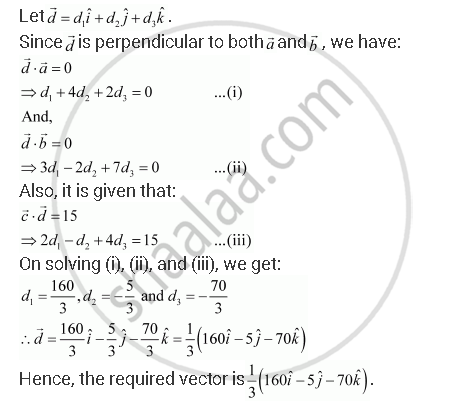Share

# Let Veca = Hati + 4hatj + 2hatk, Vecb = 3hati - 2hatj + 7hatk  and Vecc = 2hati - Hatj + 4hatk. Find a Vector Vecd Which is Perpendicular to Both Veca and Vecb, and Vecc.Vecd = 15. - CBSE (Commerce) Class 12 - Mathematics

#### Question

Let veca = hati + 4hatj + 2hatk, vecb = 3hati - 2hatj + 7hatk  and vecc = 2hati - hatj + 4hatk. Find a vector vecd which is perpendicular to both veca and vecb, and vecc.vecd = 15.

#### SolutionIs there an error in this question or solution?

#### Video TutorialsVIEW ALL 

Solution Let Veca = Hati + 4hatj + 2hatk, Vecb = 3hati - 2hatj + 7hatk  and Vecc = 2hati - Hatj + 4hatk. Find a Vector Vecd Which is Perpendicular to Both Veca and Vecb, and Vecc.Vecd = 15. Concept: Addition of Vectors.
S